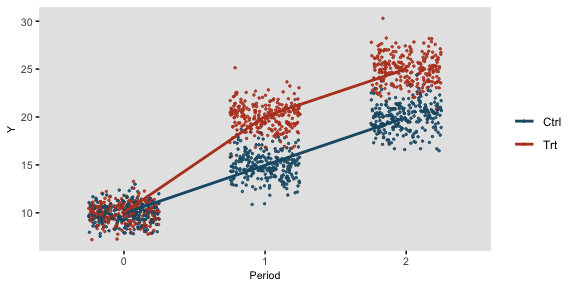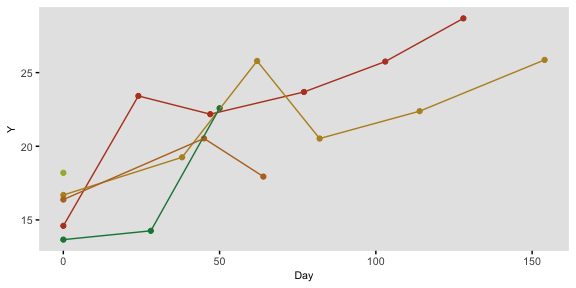# Longitudinal Data

To simulate longitudinal data, we start with a ‘cross-sectional’ data set and convert it to a time-dependent data set. The original cross-sectional data set may or may not include time-dependent data in the columns. In the next example, we measure outcome Y once before and twice after intervention T in a randomized trial:

tdef <- defData(varname = "T", dist = "binary", formula = 0.5)
tdef <- defData(tdef, varname = "Y0", dist = "normal", formula = 10, variance = 1)
tdef <- defData(tdef, varname = "Y1", dist = "normal", formula = "Y0 + 5 + 5 * T",
variance = 1)
tdef <- defData(tdef, varname = "Y2", dist = "normal", formula = "Y0 + 10 + 5 * T",
variance = 1)

dtTrial <- genData(500, tdef)
dtTrial
##       id T        Y0       Y1       Y2
##   1:   1 0  9.616066 14.98298 19.92275
##   2:   2 1  9.949226 21.26938 27.82876
##   3:   3 0  9.574763 16.04243 19.22452
##   4:   4 1 10.355033 22.04683 25.46194
##   5:   5 1  8.036624 18.74018 24.03711
##  ---
## 496: 496 1  9.517070 18.16391 23.86068
## 497: 497 0 11.125862 15.56157 22.52300
## 498: 498 0  9.952948 13.81096 18.13264
## 499: 499 1 10.470502 19.89448 25.32632
## 500: 500 1 11.191676 20.47816 27.01280

The data in longitudinal form is created with a call to addPeriods. If the cross-sectional data includes time dependent data, then the number of periods nPeriods must be the same as the number of time dependent columns. If a variable is not declared as one of the timevars, it will be repeated each time period. In this example, the treatment indicator T is not specified as a time dependent variable. (Note: if there are two time-dependent variables, it is best to create two data sets and merge them. This will be shown later in the vignette).

dtTime <- addPeriods(dtTrial, nPeriods = 3, idvars = "id", timevars = c("Y0", "Y1",
"Y2"), timevarName = "Y")
dtTime
##        id period T         Y timeID
##    1:   1      0 0  9.616066      1
##    2:   1      1 0 14.982980      2
##    3:   1      2 0 19.922754      3
##    4:   2      0 1  9.949226      4
##    5:   2      1 1 21.269376      5
##   ---
## 1496: 499      1 1 19.894479   1496
## 1497: 499      2 1 25.326321   1497
## 1498: 500      0 1 11.191676   1498
## 1499: 500      1 1 20.478165   1499
## 1500: 500      2 1 27.012803   1500

This is what the longitudinal data look like:### Longitudinal data with varying observation and interval times

It is also possible to generate longitudinal data with varying numbers of measurement periods as well as varying time intervals between each measurement period. This is done by defining specific variables in the data set that define the number of observations per subject and the average interval time between each observation. nCount defines the number of measurements for an individual; mInterval specifies the average time between intervals for an subject; and vInterval specifies the variance of those interval times. If vInterval is set to 0 or is not defined, the interval for a subject is determined entirely by the mean interval. If vInterval is greater than 0, time intervals are generated using a gamma distribution with mean and dispersion specified.

In this simple example, the cross-sectional data generates individuals with a different number of measurement observations and different times between each observation. Data for two of these individuals is printed:

def <- defData(varname = "xbase", dist = "normal", formula = 20, variance = 3)
def <- defData(def, varname = "nCount", dist = "noZeroPoisson", formula = 6)
def <- defData(def, varname = "mInterval", dist = "gamma", formula = 30, variance = 0.01)
def <- defData(def, varname = "vInterval", dist = "nonrandom", formula = 0.07)

dt <- genData(200, def)
dt[id %in% c(8, 121)]  # View individuals 8 and 121
##     id    xbase nCount mInterval vInterval
## 1:   8 21.88506      1  24.66097      0.07
## 2: 121 19.84156      8  31.21209      0.07

The resulting longitudinal data for these two subjects can be inspected after a call to addPeriods. Notice that no parameters need to be set since all information resides in the data set itself:

dtPeriod <- addPeriods(dt)
dtPeriod[id %in% c(8, 121)]  # View individuals 8 and 121 only
##     id period    xbase time timeID
## 1:   8      0 21.88506    0     42
## 2: 121      0 19.84156    0    728
## 3: 121      1 19.84156   25    729
## 4: 121      2 19.84156   53    730
## 5: 121      3 19.84156   75    731
## 6: 121      4 19.84156  106    732
## 7: 121      5 19.84156  128    733
## 8: 121      6 19.84156  152    734
## 9: 121      7 19.84156  186    735

If a time sensitive measurement is added to the data set …

def2 <- defDataAdd(varname = "Y", dist = "normal", formula = "15 + .1 * time", variance = 5)
dtPeriod <- addColumns(def2, dtPeriod)

… a plot of a five randomly selected individuals looks like this: